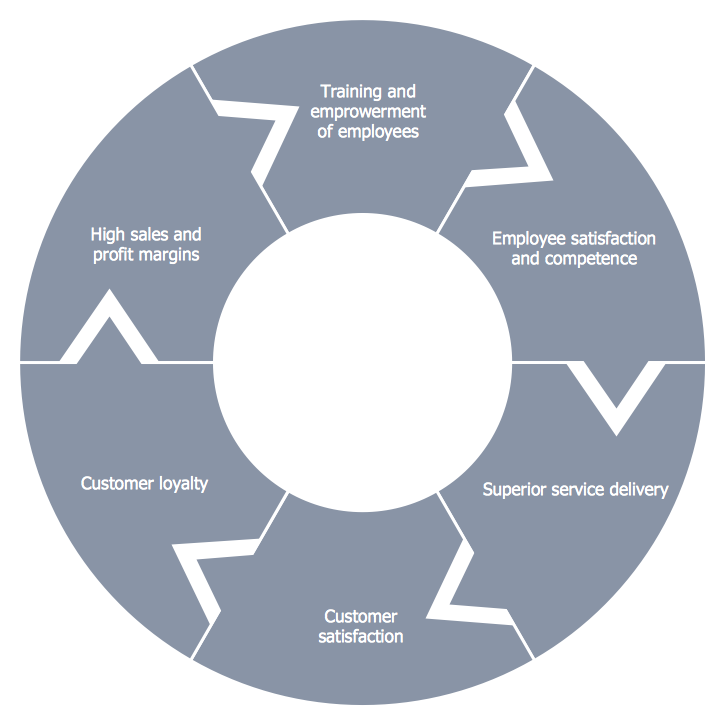This site uses cookies. By continuing to browse the ConceptDraw site you are agreeing to our Use of Site Cookies.
ConceptDraw Samples | Marketing — Target & Circular Diagrams

# Target and Circular Diagrams

Samples of Marketing Target and Circular Diagrams are created with ConceptDraw DIAGRAM diagramming and vector drawing software enhanced with Target and Circular Diagrams solution from ConceptDraw Solution Park. This solution extends ConceptDraw DIAGRAM with samples, templates and a library of design elements for drawing Target and Circular Diagrams; including - Target Diagrams, Brand Essence Model Diagram, Daily Schedule Chart, Macro-Micro Environmental Forces Diagram, Market Scope Diagram, Marketing Mix Diagram, Ring Chart, Stakeholder Diagram.

ConceptDraw DIAGRAM provides export of vector graphic multipage documents into multiple file formats: vector graphics (SVG, EMF, EPS), bitmap graphics (PNG, JPEG, GIF, BMP, TIFF), web documents (HTML, PDF), PowerPoint presentations (PPT), Adobe Flash (SWF).

## Tutorials and Solutions:

Video Tutorials: ConceptDraw Solution Park

Solutions: Target and Circular Diagrams for ConceptDraw DIAGRAM

## Sample 1: Circular Diagram – The Content Marketing Cycle

Target and Circular Diagrams sample: Circular diagram – The content marketing cycle

This example is created using ConceptDraw DIAGRAM diagramming and vector drawing software enhanced with Target and Circular Diagrams solution from ConceptDraw Solution Park.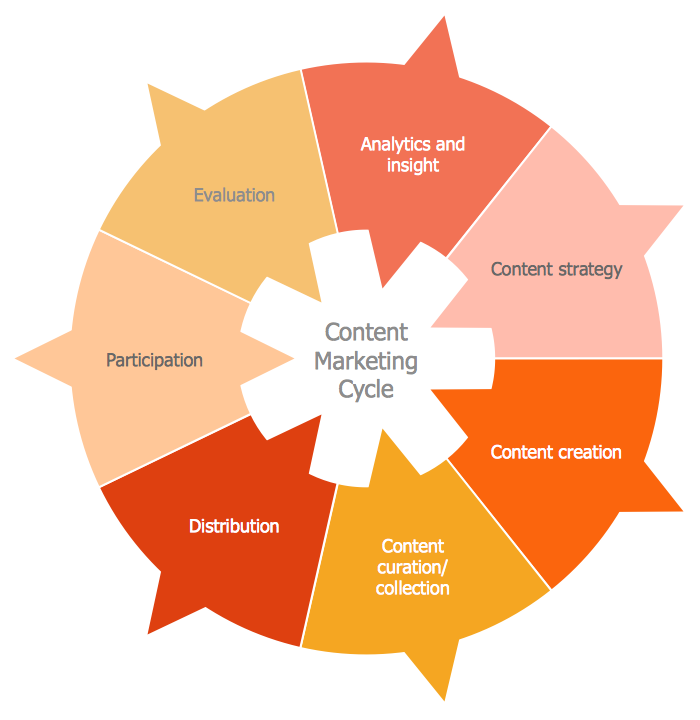## Sample 2: Circular Diagram – Vicious Circle

Target and Circular Diagrams sample: Circular diagram – Vicious circle.

This example is created using ConceptDraw DIAGRAM diagramming and vector drawing software enhanced with Target and Circular Diagrams solution from ConceptDraw Solution Park.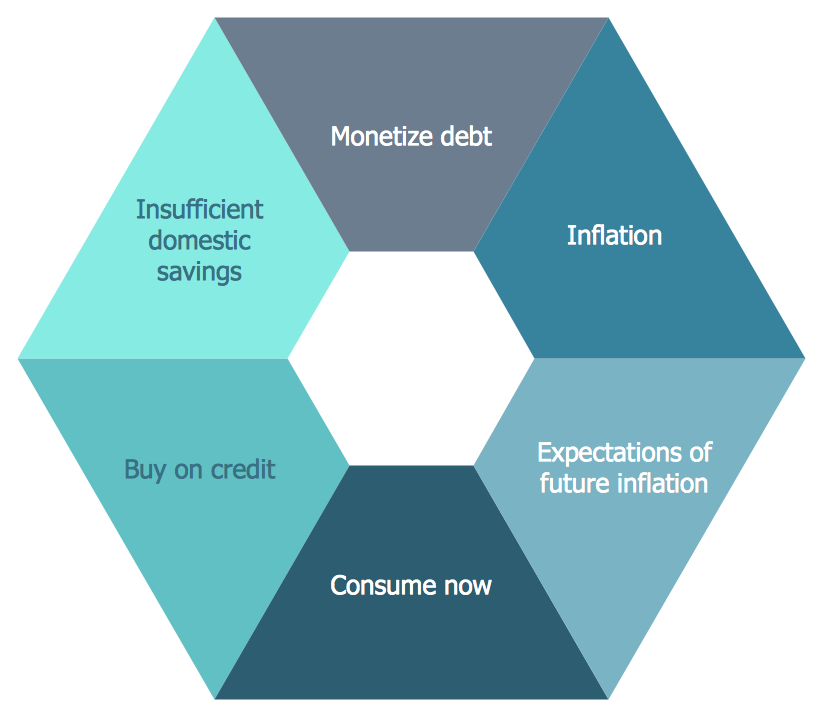## Sample 3: Pie Chart – Energy Consumption

Target and Circular Diagrams sample: Circular diagram – Energy consumption.

This example is created using ConceptDraw DIAGRAM diagramming and vector drawing software enhanced with Target and Circular Diagrams solution from ConceptDraw Solution Park.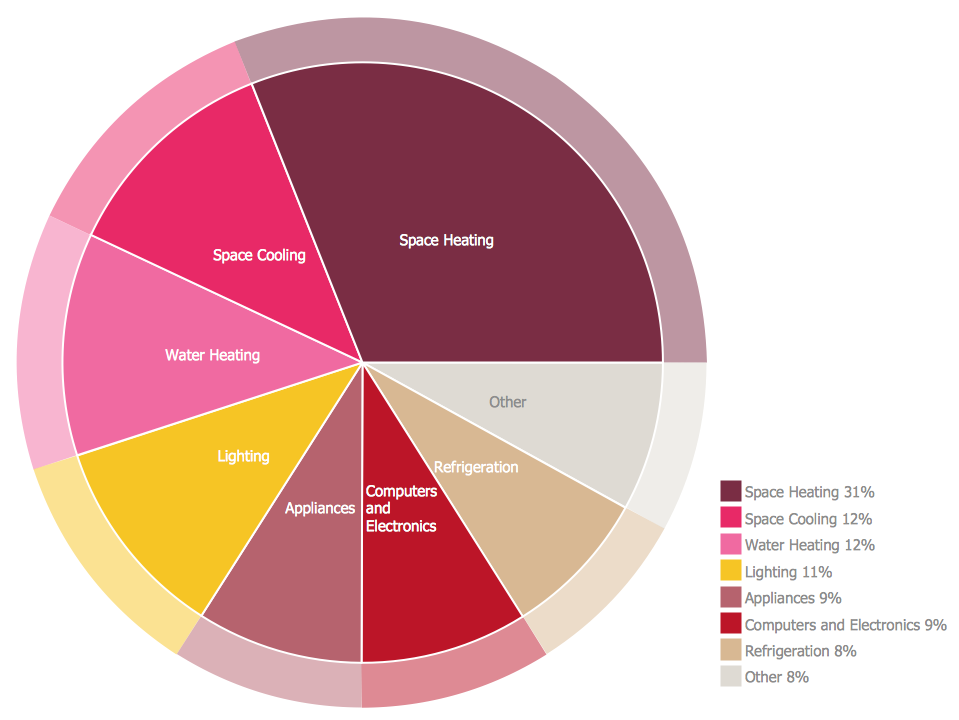## Sample 4: Schedule Chart – Daily Action Plan

Target and Circular Diagrams sample: Schedule chart – Daily action plan.

This example is created using ConceptDraw DIAGRAM diagramming and vector drawing software enhanced with Target and Circular Diagrams solution from ConceptDraw Solution Park.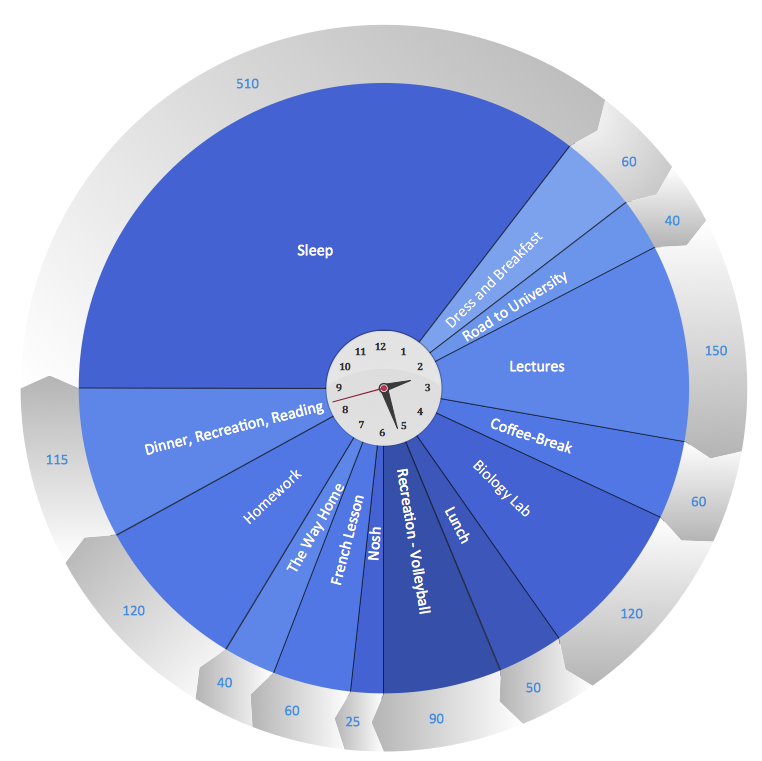## Sample 5: Circular Diagram – Planning Cycle

Target and Circular Diagrams sample: Circular diagram – Planning cycle.

This example is created using ConceptDraw DIAGRAM diagramming and vector drawing software enhanced with Target and Circular Diagrams solution from ConceptDraw Solution Park.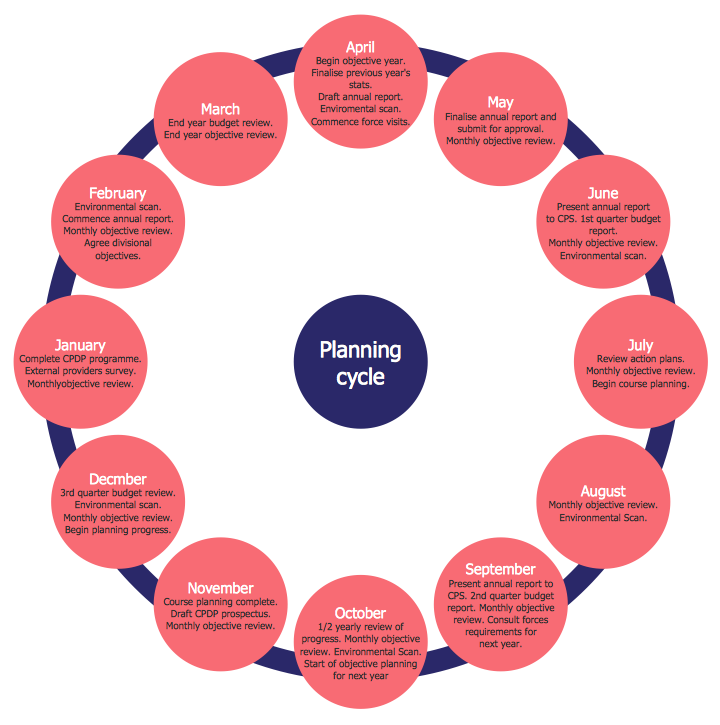## Sample 6: Target Diagram – Brand Essence Model

Target and Circular Diagrams sample: Target diagram - Brand essence model.

This example is created using ConceptDraw DIAGRAM diagramming and vector drawing software enhanced with Target and Circular Diagrams solution from ConceptDraw Solution Park.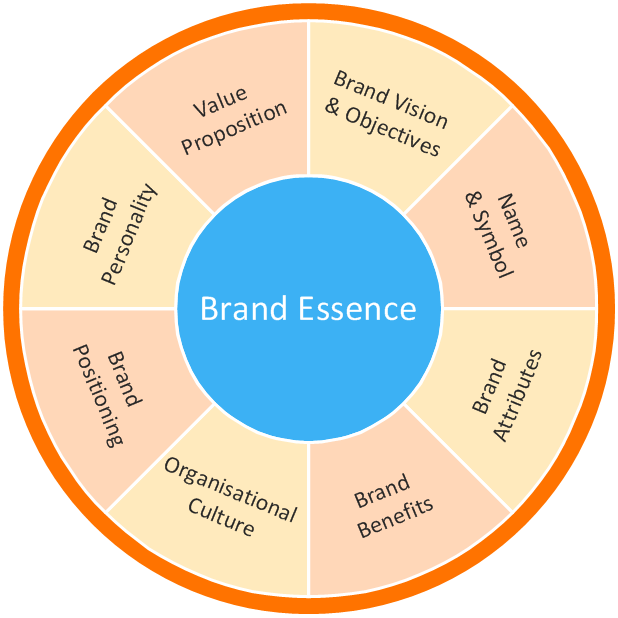## Sample 7: Target Diagram – Foreign Trade

Target and Circular Diagrams sample: Target diagram - Foreign trade.

This example is created using ConceptDraw DIAGRAM diagramming and vector drawing software enhanced with Target and Circular Diagrams solution from ConceptDraw Solution Park.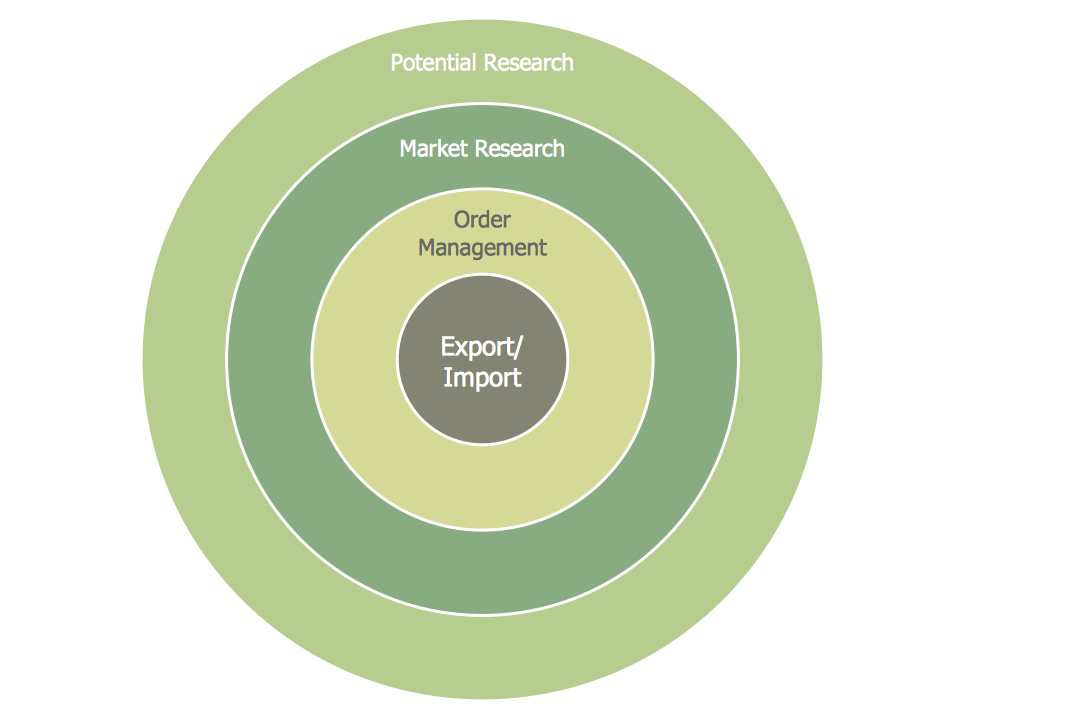## Sample 8: Diagram – Target Market

Target and Circular Diagrams sample: Diagram – Target market.

This example is created using ConceptDraw DIAGRAM diagramming and vector drawing software enhanced with Target and Circular Diagrams solution from ConceptDraw Solution Park.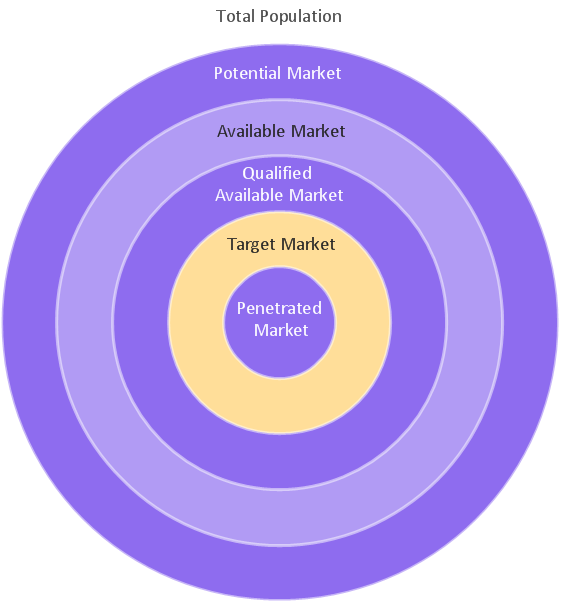## Sample 9: A Framework for Understanding and Improving Interactions

Target and Circular Diagrams sample: A framework for understanding and improving interactions.

This example is created using ConceptDraw DIAGRAM diagramming and vector drawing software enhanced with Target and Circular Diagrams solution from ConceptDraw Solution Park.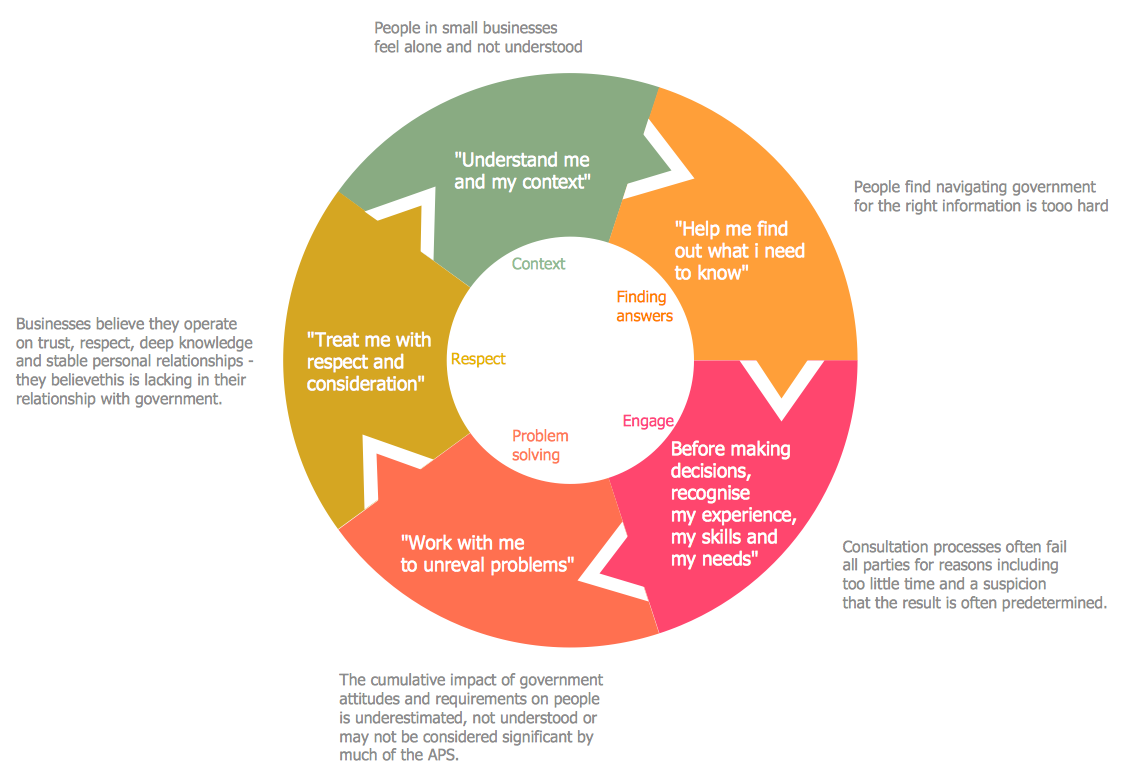## Sample 10: Circular Diagram – Assessment

Target and Circular Diagrams sample: Circular diagram – Assessment.

This example is created using ConceptDraw DIAGRAM diagramming and vector drawing software enhanced with Target and Circular Diagrams solution from ConceptDraw Solution Park.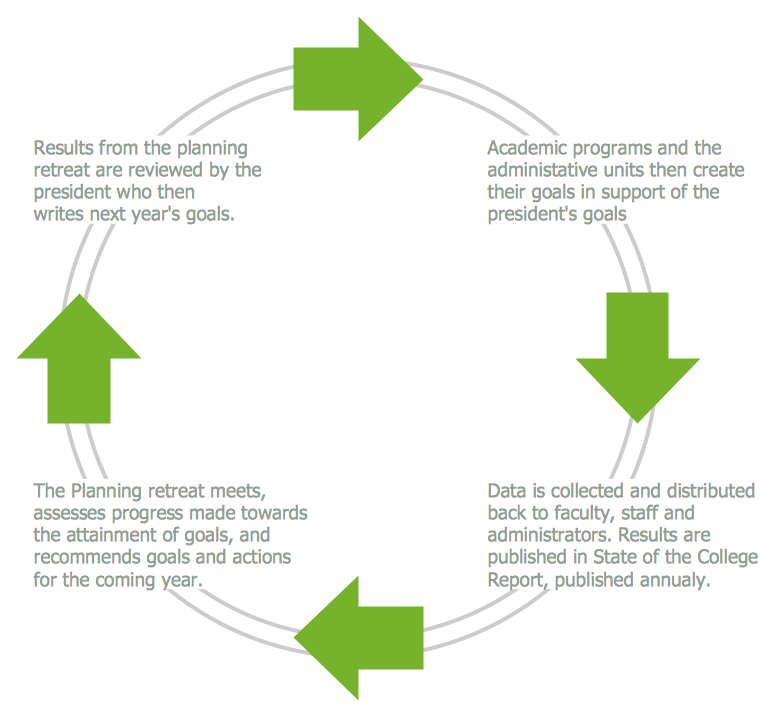## Sample 11: Circular Diagram – Business Feedback

Target and Circular Diagrams sample: Circular diagram – Business feedback.

This example is created using ConceptDraw DIAGRAM diagramming and vector drawing software enhanced with Target and Circular Diagrams solution from ConceptDraw Solution Park.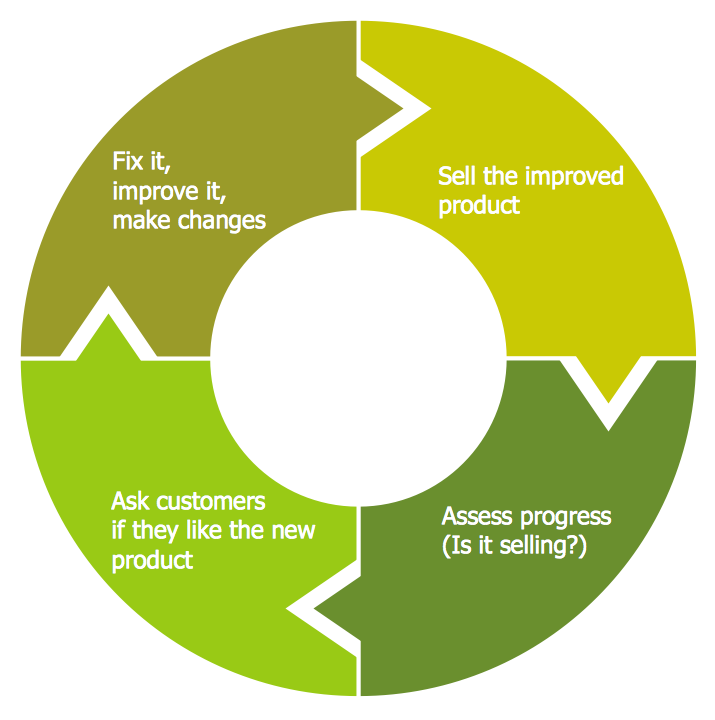## Sample 12: Circular Diagram – Collaboration Life Cicle

Target and Circular Diagrams sample: Circular diagram – Collaboration life cicle.

This example is created using ConceptDraw DIAGRAM diagramming and vector drawing software enhanced with Target and Circular Diagrams solution from ConceptDraw Solution Park.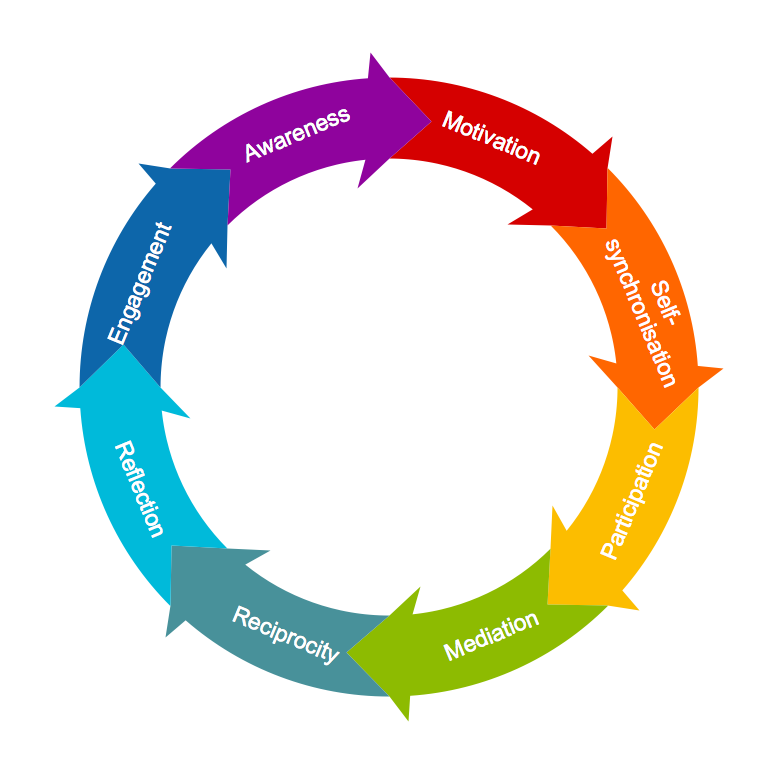## Sample 13: Circular Diagram – Community Life Cycle

Target and Circular Diagrams sample: Circular diagram – Community life cycle.

This example is created using ConceptDraw DIAGRAM diagramming and vector drawing software enhanced with Target and Circular Diagrams solution from ConceptDraw Solution Park.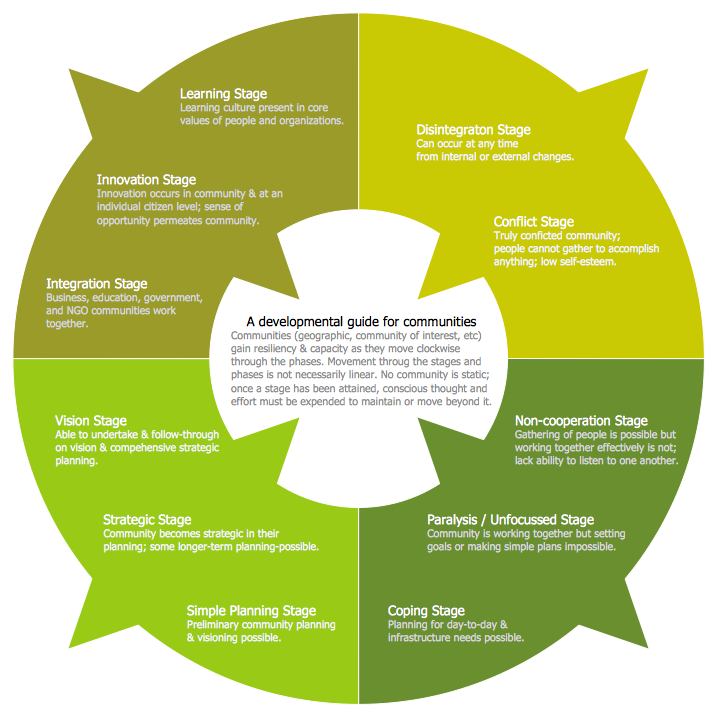## Sample 14: Target Diagram – Content Marketing Cycle

Target and Circular Diagrams sample: Target diagram – Content marketing cycle.

This example is created using ConceptDraw DIAGRAM diagramming and vector drawing software enhanced with Target and Circular Diagrams solution from ConceptDraw Solution Park.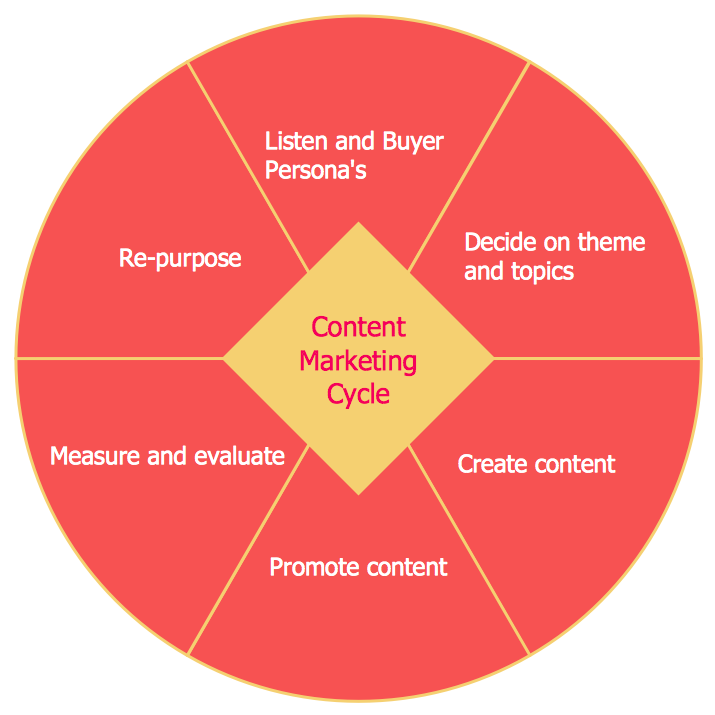## Sample 15: Circular Diagram – Cultural Competence

Target and Circular Diagrams sample: Circular diagram – Cultural competence.

This example is created using ConceptDraw DIAGRAM diagramming and vector drawing software enhanced with Target and Circular Diagrams solution from ConceptDraw Solution Park.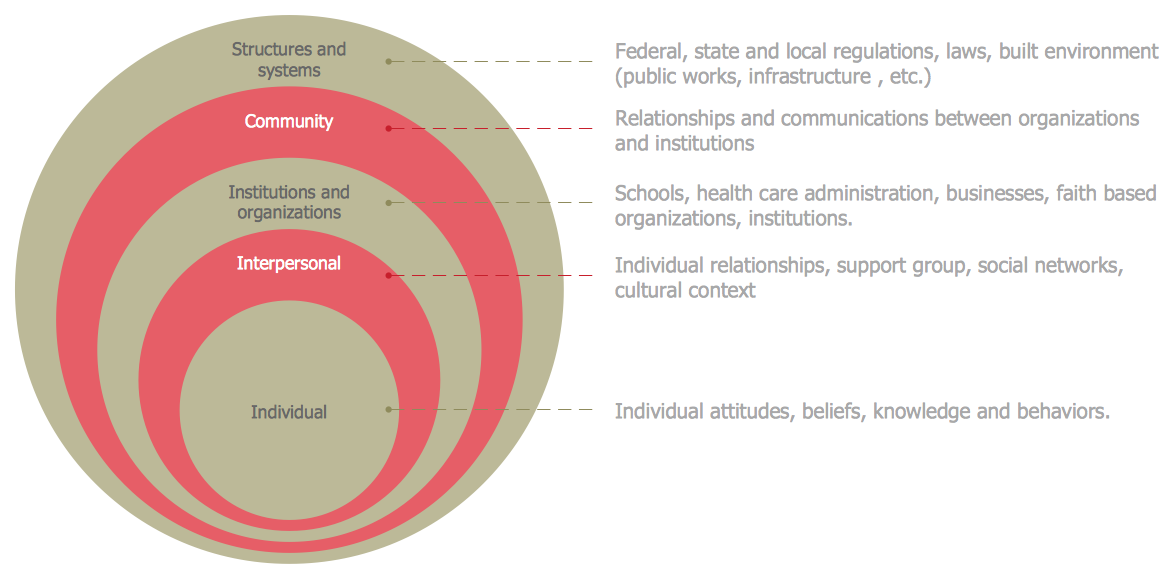## Sample 16: Circular Diagram – Cycle

Target and Circular Diagrams sample: Circular diagram – Cycle.

This example is created using ConceptDraw DIAGRAM diagramming and vector drawing software enhanced with Target and Circular Diagrams solution from ConceptDraw Solution Park.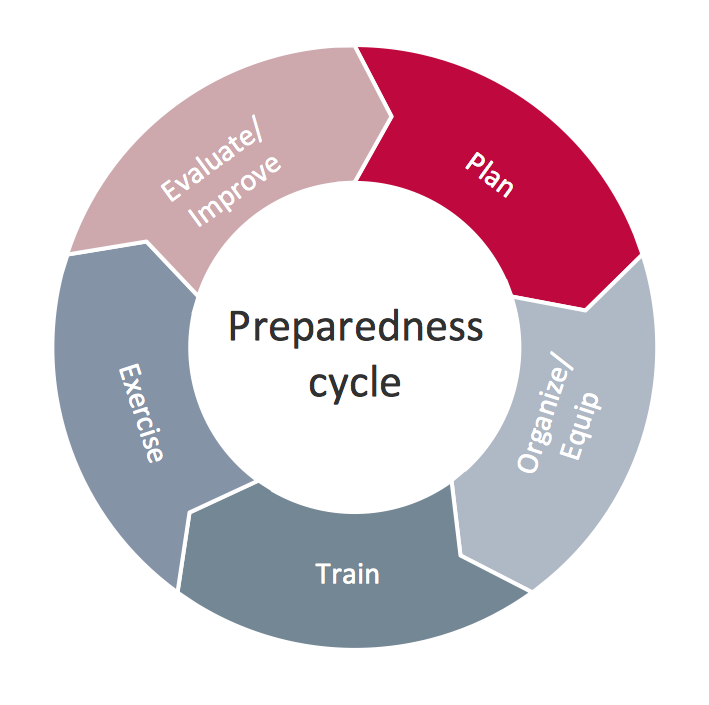## Sample 17: Target Digram – Cycle of Automobile Dependency

Target and Circular Diagrams sample: Target digram – Cycle of automobile dependency.

This example is created using ConceptDraw DIAGRAM diagramming and vector drawing software enhanced with Target and Circular Diagrams solution from ConceptDraw Solution Park.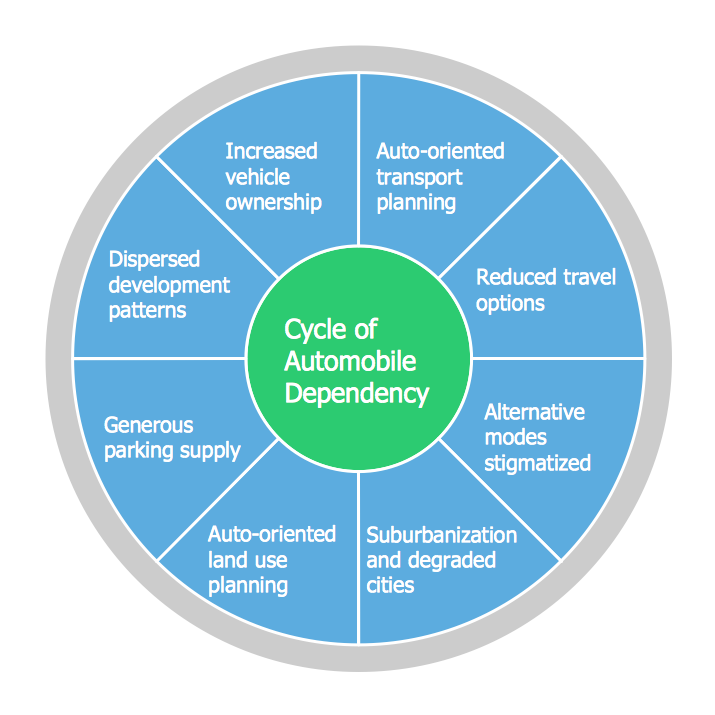## Sample 18: Target Diagram – Brand Essence Model

Target and Circular Diagrams sample: Target diagram – Brand essence model.

This example is created using ConceptDraw DIAGRAM diagramming and vector drawing software enhanced with Target and Circular Diagrams solution from ConceptDraw Solution Park.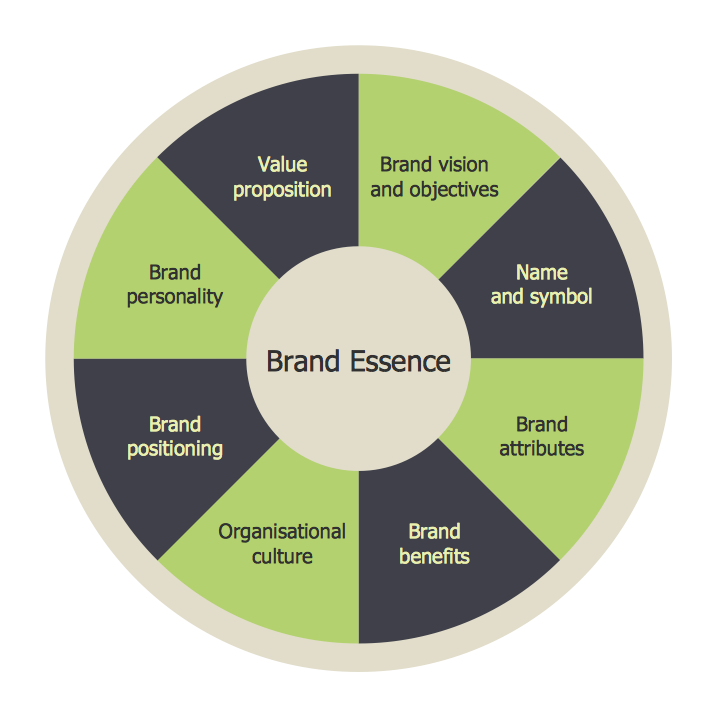## Sample 19: Diagram – Target Market

Target and Circular Diagrams sample: Diagram – Target market.

This example is created using ConceptDraw DIAGRAM diagramming and vector drawing software enhanced with Target and Circular Diagrams solution from ConceptDraw Solution Park.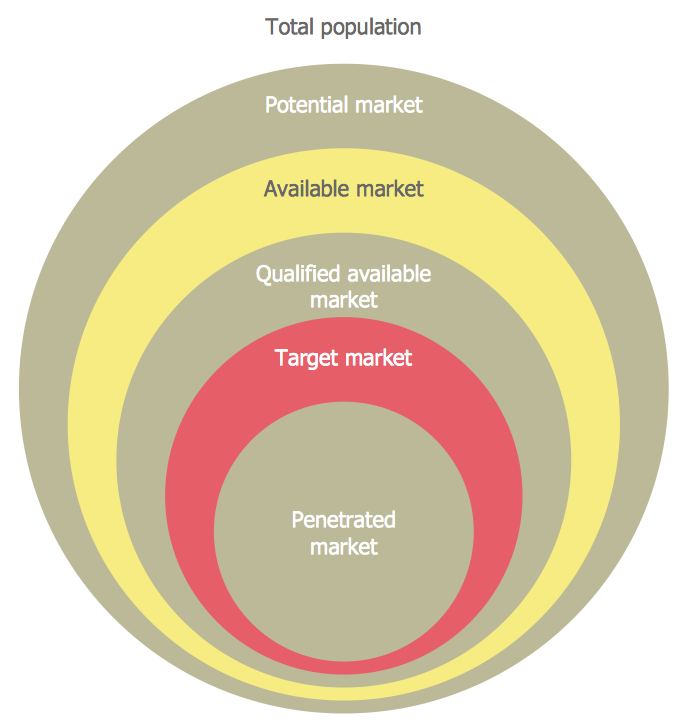## Sample 20: Circular Diagram – Emission Avoidance

Target and Circular Diagrams sample: Circular diagram – Emission avoidance.

This example is created using ConceptDraw DIAGRAM diagramming and vector drawing software enhanced with Target and Circular Diagrams solution from ConceptDraw Solution Park.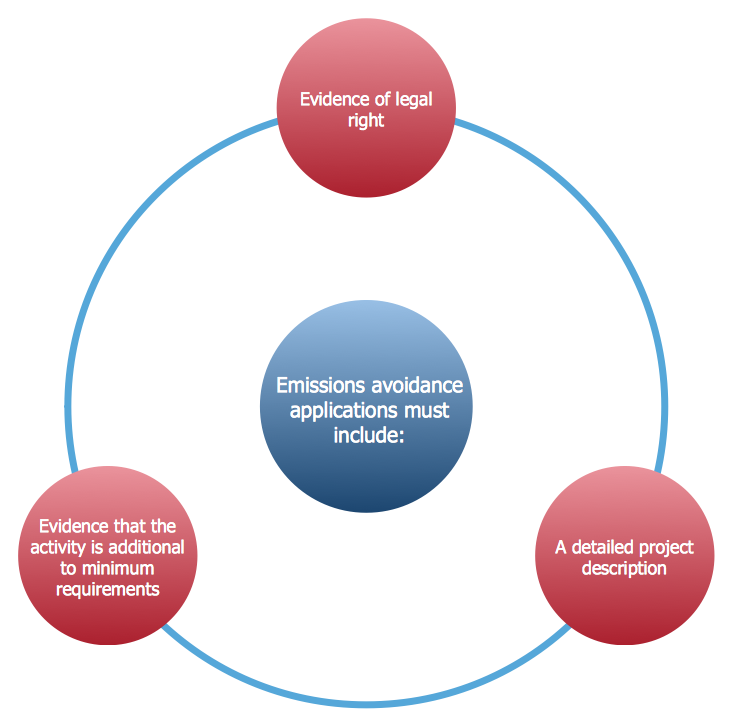## Sample 21: Circular Diagrams Forces Shaping the Broadband Ecosystem in the United States

Target and Circular Diagrams sample: Circular diagrams forces shaping the broadband ecosystem in the united states.

This example is created using ConceptDraw DIAGRAM diagramming and vector drawing software enhanced with Target and Circular Diagrams solution from ConceptDraw Solution Park.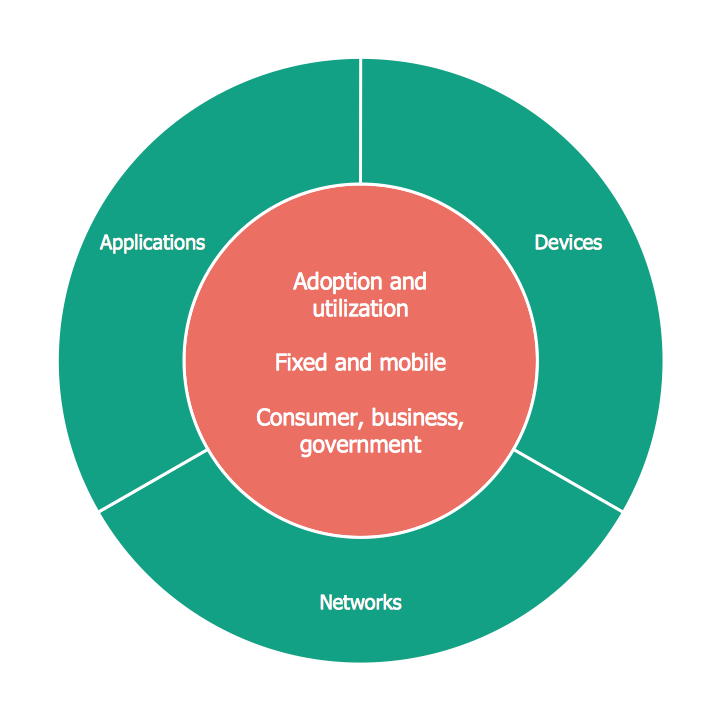## Sample 22: Circular Diagram – Grant Life Cycle

Target and Circular Diagrams sample: Circular diagram – Grant life cycle

This example is created using ConceptDraw DIAGRAM diagramming and vector drawing software enhanced with Target and Circular Diagrams solution from ConceptDraw Solution Park.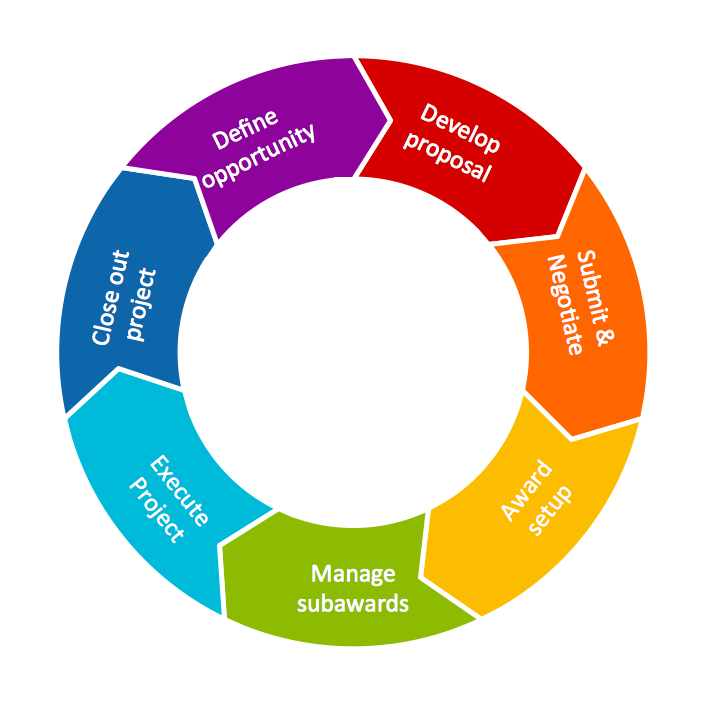## Sample 23: Circular Diagram – Life as an Intelligence Analyst

Target and Circular Diagrams sample: Circular diagram – Life as an intelligence analyst.

This example is created using ConceptDraw DIAGRAM diagramming and vector drawing software enhanced with Target and Circular Diagrams solution from ConceptDraw Solution Park.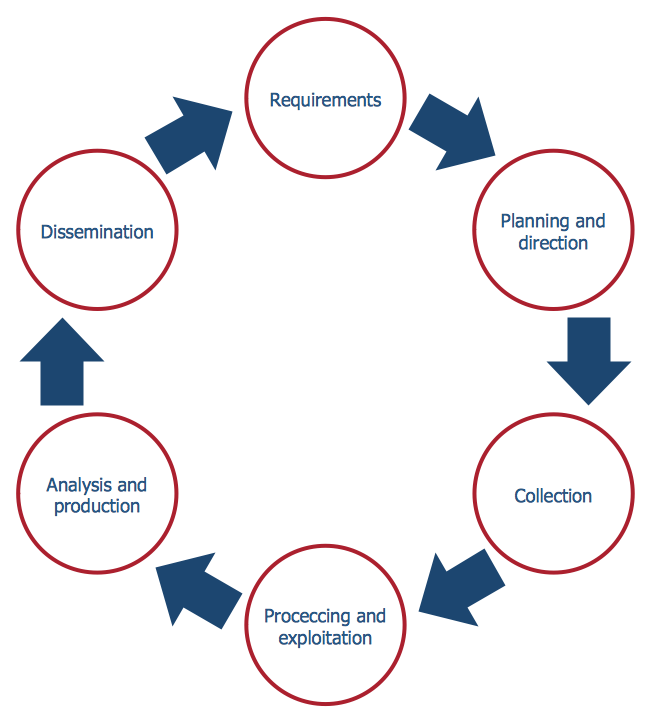## Sample 24: Circular Diagram – Life Cycle Analysis

Target and Circular Diagrams sample: Circular diagram – Life cycle analysis.

This example is created using ConceptDraw DIAGRAM diagramming and vector drawing software enhanced with Target and Circular Diagrams solution from ConceptDraw Solution Park.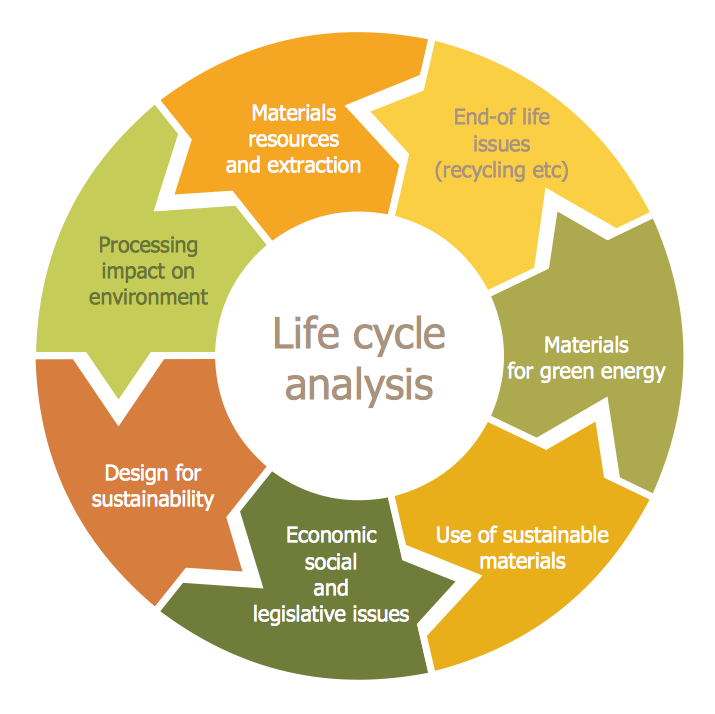## Sample 25: Circular Diagram – Loyality

Target and Circular Diagrams sample: Circular diagram – Loyality.

This example is created using ConceptDraw DIAGRAM diagramming and vector drawing software enhanced with Target and Circular Diagrams solution from ConceptDraw Solution Park.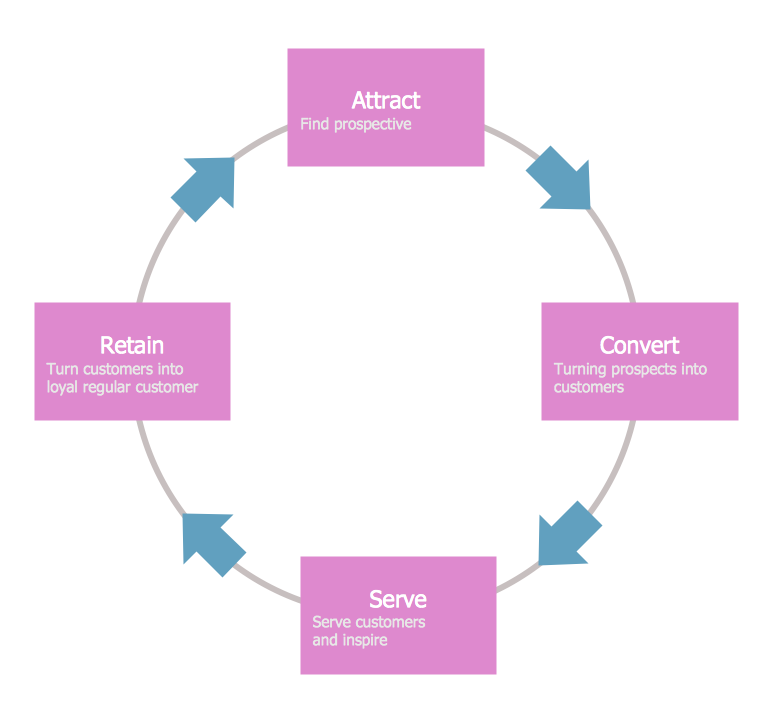## Sample 26: Target Diagram – Marketing Mix Wheel

Target and Circular Diagrams sample: Target diagram – Marketing mix wheel.

This example is created using ConceptDraw DIAGRAM diagramming and vector drawing software enhanced with Target and Circular Diagrams solution from ConceptDraw Solution Park.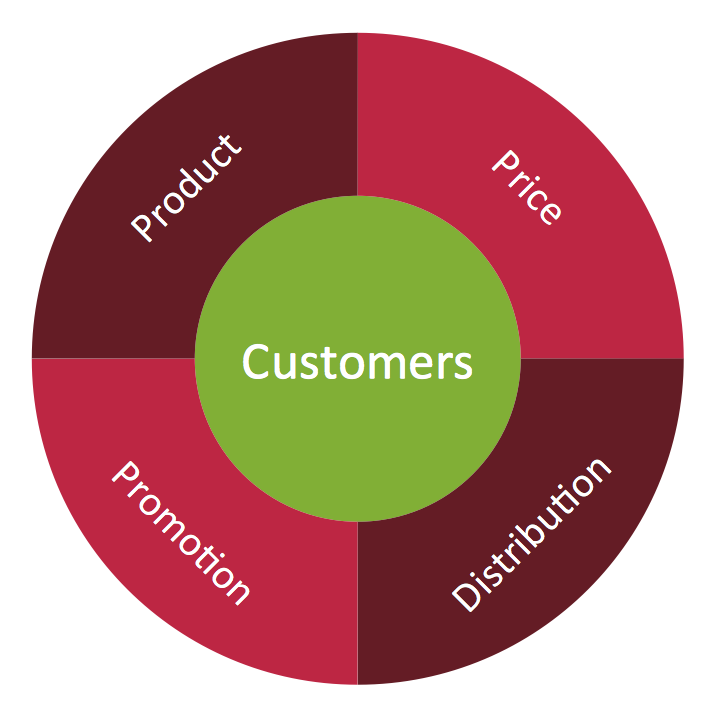## Sample 27: Circular Diagram – Post Award Process

Target and Circular Diagrams sample: Circular diagram – Post award process.

This example is created using ConceptDraw DIAGRAM diagramming and vector drawing software enhanced with Target and Circular Diagrams solution from ConceptDraw Solution Park.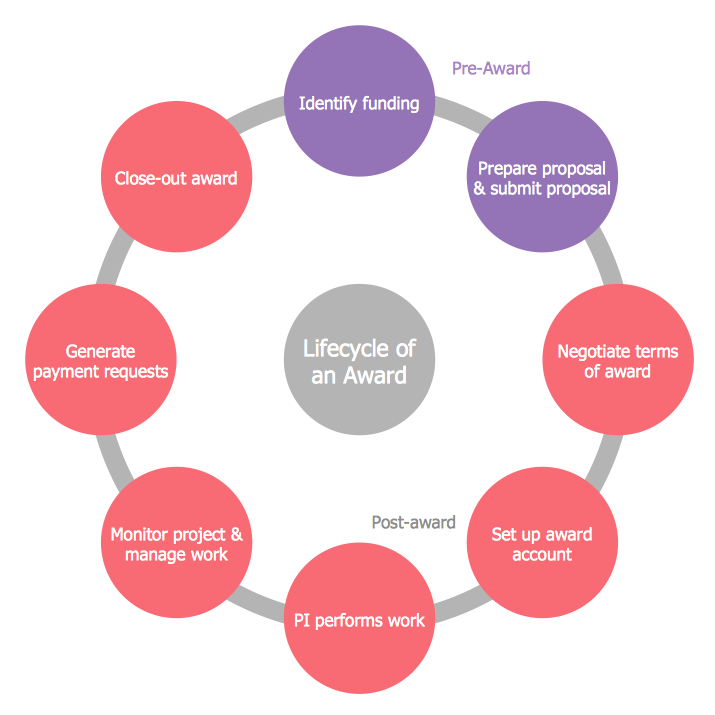## Sample 28: Circular Diagram – Research Cycle Diagram

Target and Circular Diagrams sample: Circular diagram – Research cycle diagram.

This example is created using ConceptDraw DIAGRAM diagramming and vector drawing software enhanced with Target and Circular Diagrams solution from ConceptDraw Solution Park.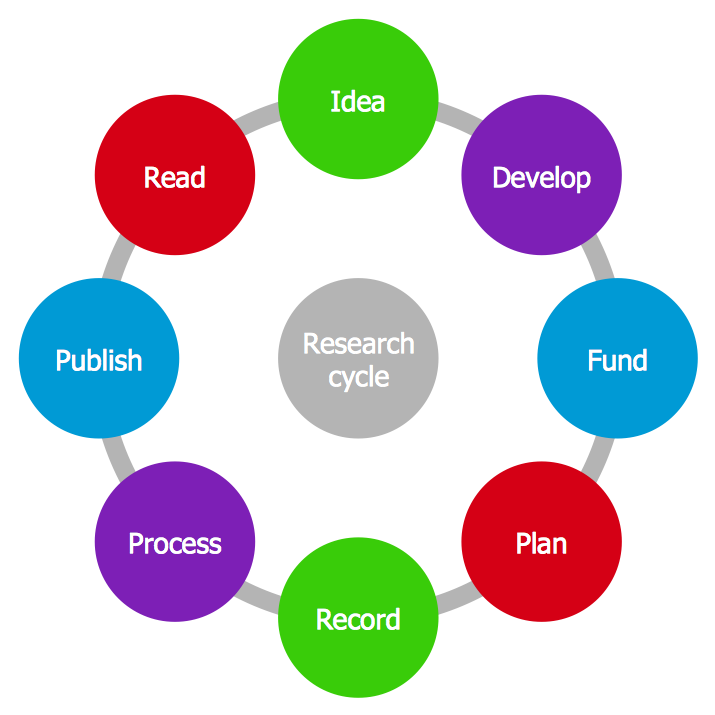## Sample 29: Circular Diagram – Strategic Planning Cycle

Target and Circular Diagrams sample: Circular diagram – Strategic planning cycle.

This example is created using ConceptDraw DIAGRAM diagramming and vector drawing software enhanced with Target and Circular Diagrams solution from ConceptDraw Solution Park.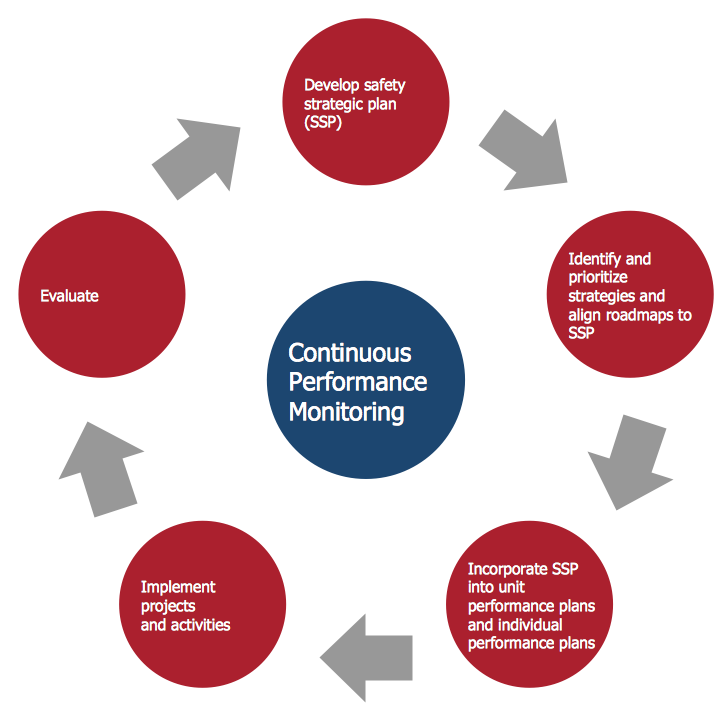## Sample 30: Circular Diagram – The Innovation Life Cycle

Target and Circular Diagrams sample: Circular diagram – The innovation life cycle.

This example is created using ConceptDraw DIAGRAM diagramming and vector drawing software enhanced with Target and Circular Diagrams solution from ConceptDraw Solution Park.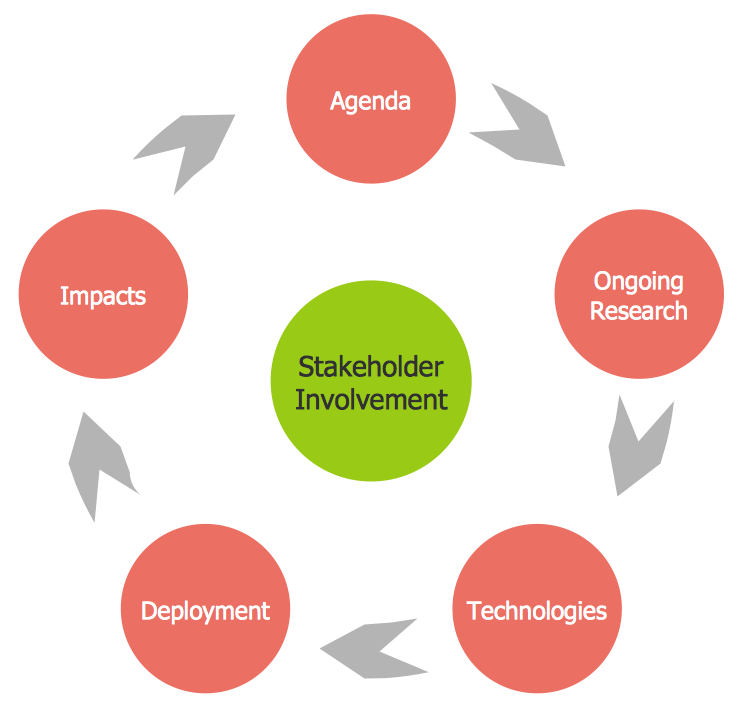## Sample 31: Target Diagram – The Marketing Plan

Target and Circular Diagrams sample: Target diagram – The marketing plan.

This example is created using ConceptDraw DIAGRAM diagramming and vector drawing software enhanced with Target and Circular Diagrams solution from ConceptDraw Solution Park.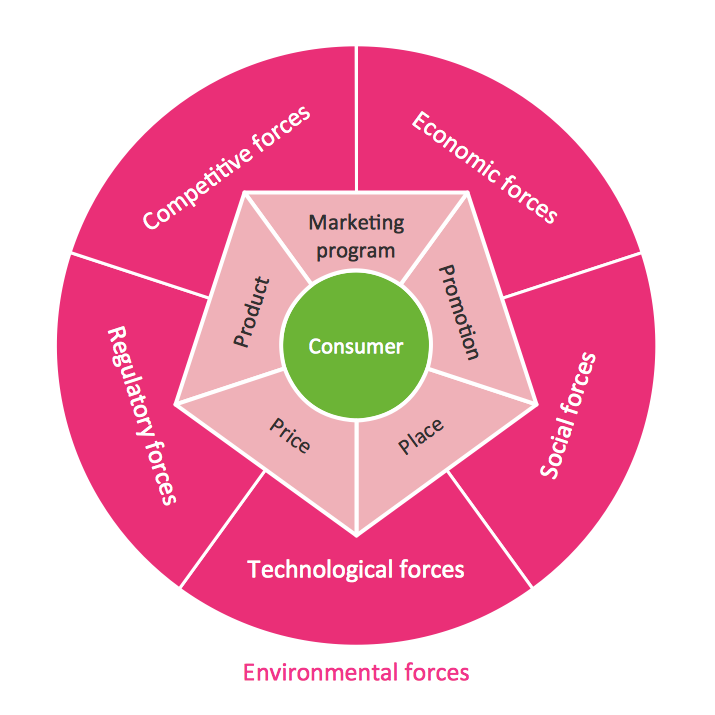## Sample 32: Circular Diagrams – Virtuous Circle (Macroeconomics)

Target and Circular Diagrams sample: Circular diagrams – Virtuous circle (macroeconomics).

This example is created using ConceptDraw DIAGRAM diagramming and vector drawing software enhanced with Target and Circular Diagrams solution from ConceptDraw Solution Park.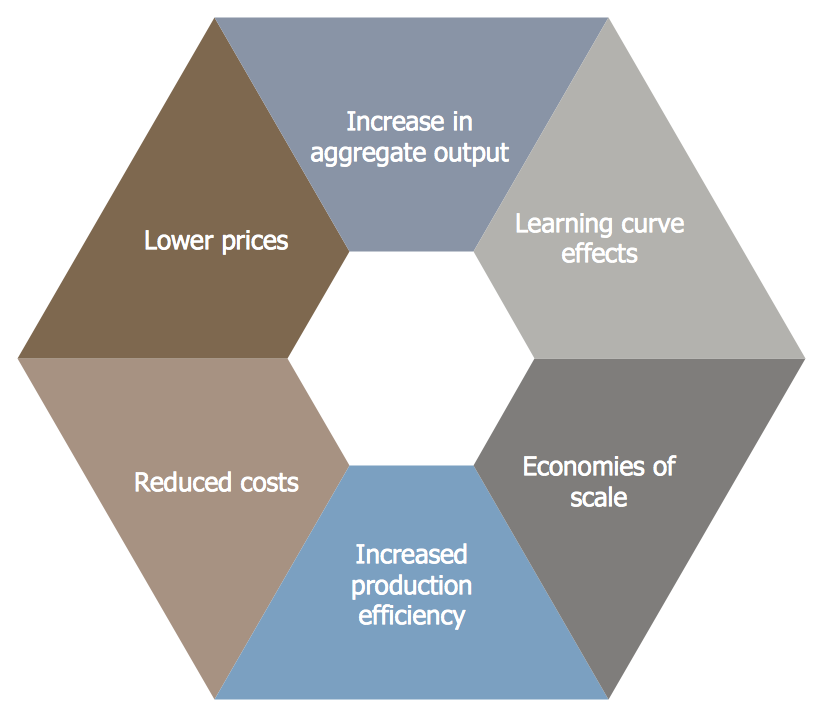## Sample 33: Circular Diagrams – Virtuous Circle (Management)

Target and Circular Diagrams sample: Circular diagrams – Virtuous circle (management).

This example is created using ConceptDraw DIAGRAM diagramming and vector drawing software enhanced with Target and Circular Diagrams solution from ConceptDraw Solution Park.